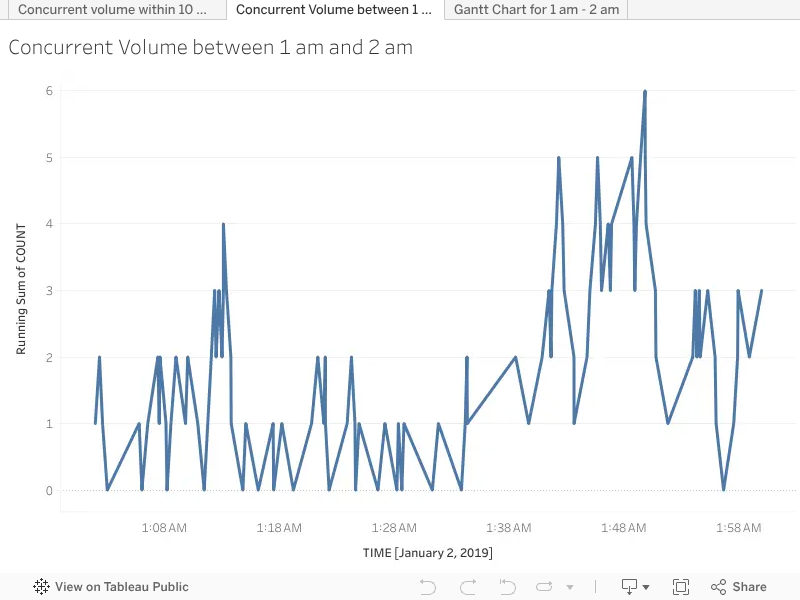2 Replies Latest reply on Jan 17, 2019 4:15 AM by Jim Dehner

# Calculating Max Concurrent Volume within 15 mins intervals

I have data where we can see each interaction of a customer along with the start time and end time of the interaction. If I count each start as 1 and each end as -1 and then tally the total over a time axis, I can see the concurrent calls using Table Functions.

Now my question is, how can I turn this into a KPI called max concurrent volume? To clarify, Maximum Concurrent Calls is not a call volume count; it is the maximum number of calls occurring simultaneously at any given second within the 15-minute time interval. So the idea is to calculate concurrent volume per second and then take the max per 15 mins window. How do I turn this concept into a Tableau calculation?• ###### 1. Re: Calculating Max Concurrent Volume within 15 mins intervals

Is there any chance to get a sample mock up data in packaged workbook?

• ###### 2. Re: Calculating Max Concurrent Volume within 15 mins intervals

Not sure I totally follow - it would be easier if you included a twbx workbook thie the data - but you are using table calculations in your analysis - they are on the bottom of the order of operations (the last calculations made - even after totals) - so what does that mean - at that point only other table calculations are available (you can nest table calcs)  - you could try window_sum() if you are still totalyying in your intervals or window_max() if yo are looking for the max

There may also be a way to avoid the table calculation in your original interval calculation using LOD's but I would need to see the data and viz

Jim

If this posts assists in resolving the question, please mark it helpful or as the 'correct answer' if it resolves the question. This will help other users find the same answer/resolution.  Thank you.